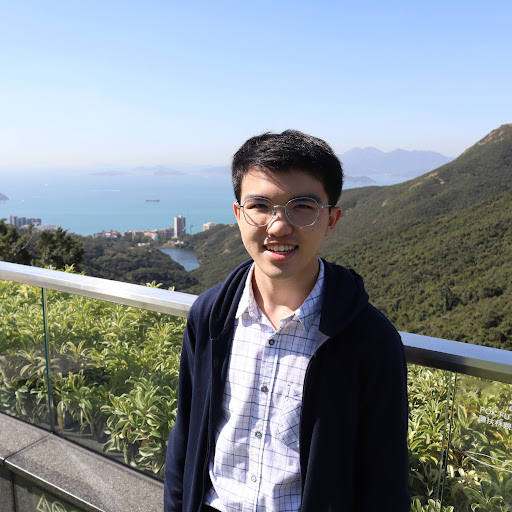# Contagion Buster - Contact Tracing with App Integration

An integration of hardware, software and applications to help alert, connect, control and reduce the spread of COVID-19.

IntermediateFull instructions provided2 days49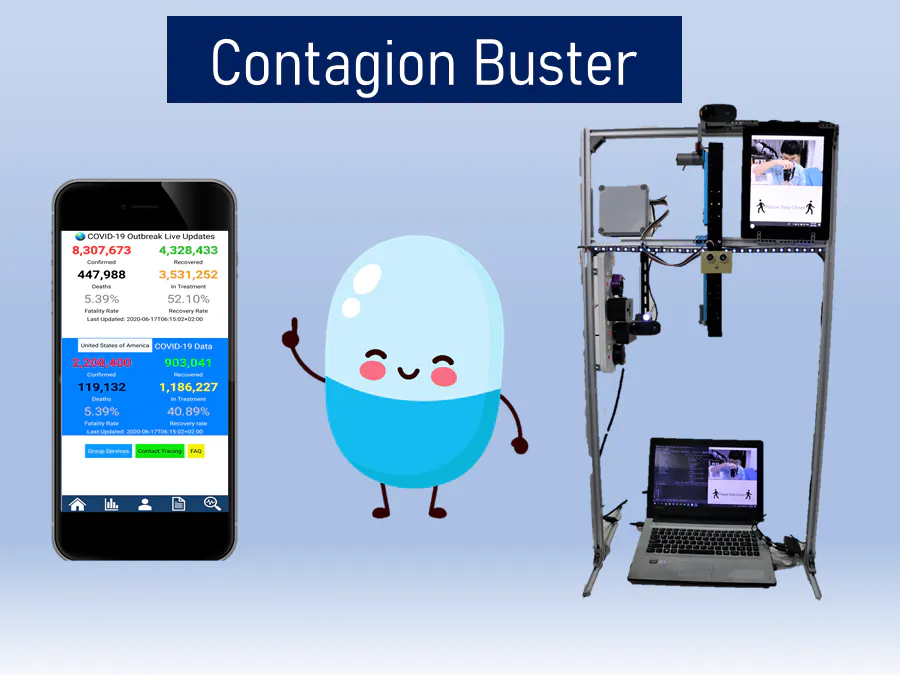## Things used in this project

### Hardware componentsArduino UNO & Genuino UNO
×1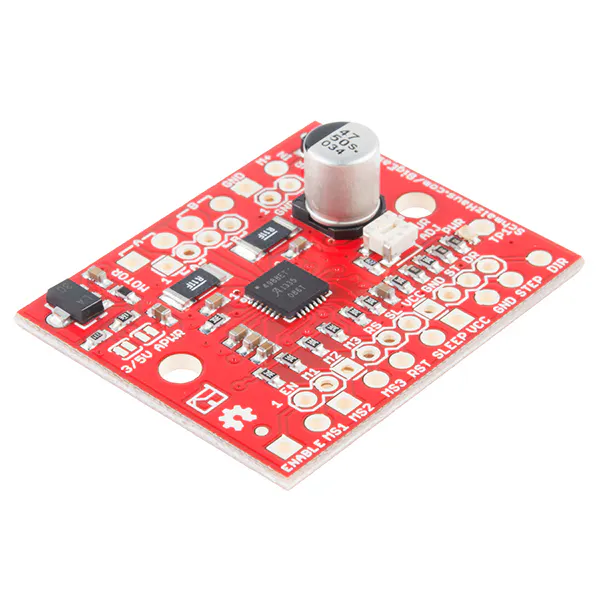SparkFun Stepper motor driver board A4988
×1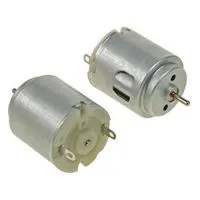Development Kit Accessory, DC Motor
×1Ultrasonic Sensor - HC-SR04 (Generic)
×1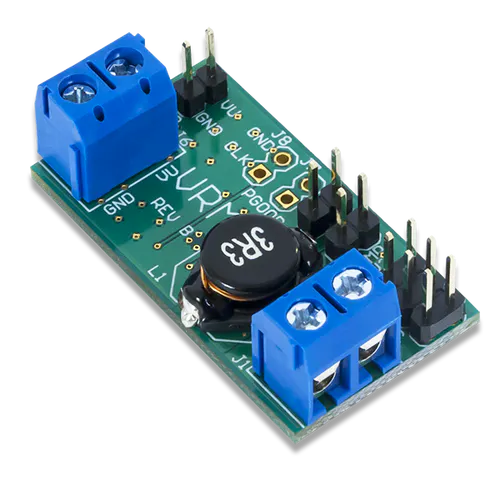Digilent Voltage Regulator Module
×1Microswitch, IP67
×1
×1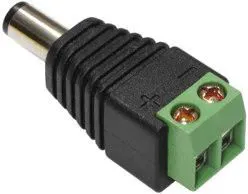Connector Adapter, DC Power - 2.1mm
×1Digilent 60W PCIe 12V 5A Power Supply
×1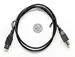USB-A to B Cable
×1
 T-Slot Aluminium
×6
 MakeBlock 80 teeth gear
×1
 MakeBlock Track with Track Axle
×1
 Cytron Technologies MLX90614 (GY-906) Infrared Thermometer Sensor
×1

### Software apps and online services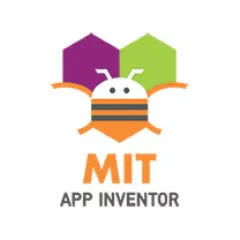MIT App InventorArduino IDE
 Jetbrains Pycharm Community

### Hand tools and fabrication machinesMultitool, ScrewdriverDrill / Driver, CordlessSoldering iron (generic)

## Schematics

### Arduino Circuit - Health Checkpoint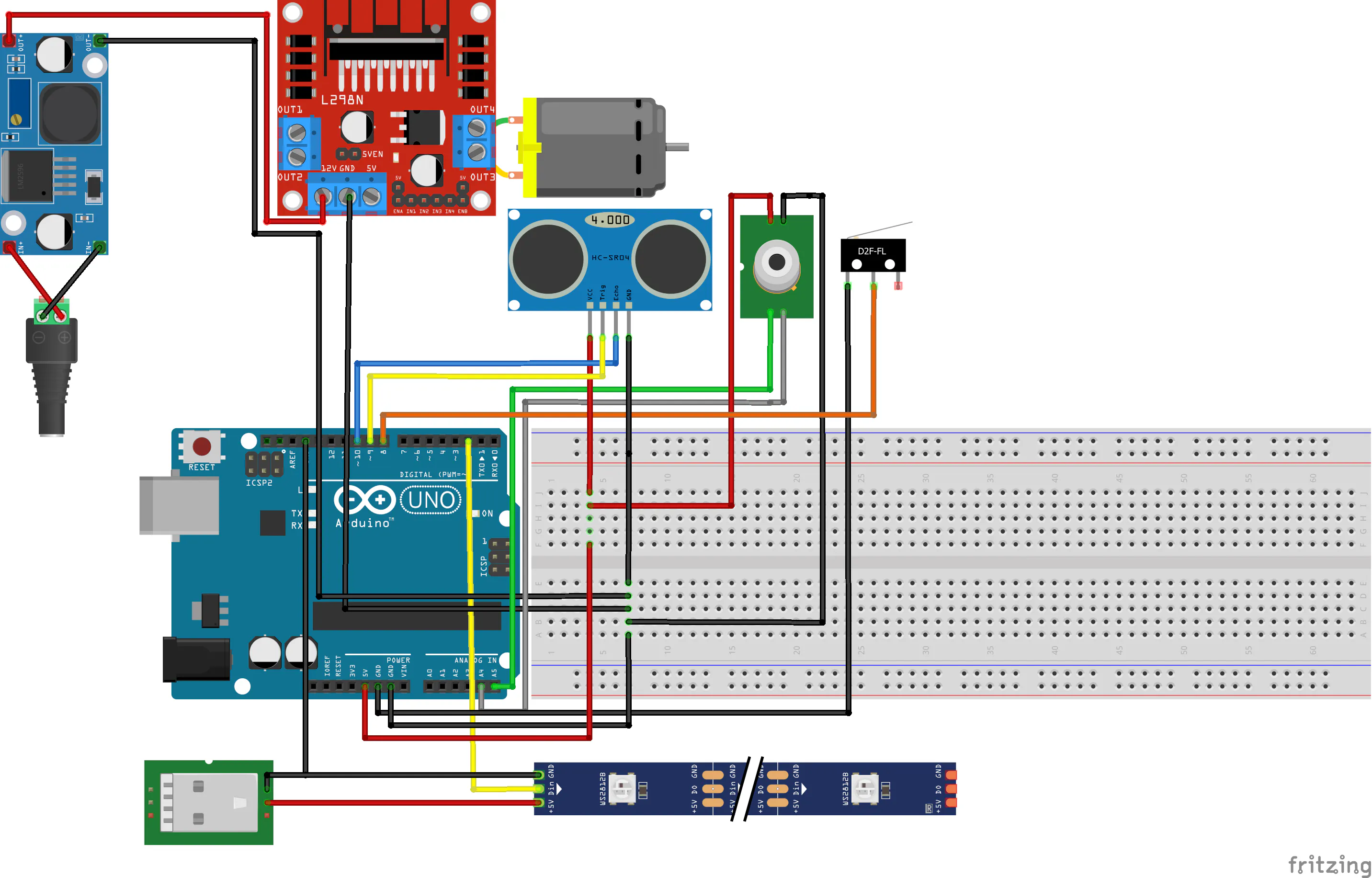## Code

### Shop Services - Health Checkpoint Python Code

Python
```import cv2
import qrcode
import serial
import time
import numpy as np

position = 0
newpos = 0

cap = cv2.VideoCapture(2)
cv2.namedWindow("frame", 0)

#set up serial
degree_sign = u"\N{DEGREE SIGN}"
ser = serial.Serial('COM21' , 9600)
time.sleep(2)

font = cv2.FONT_HERSHEY_DUPLEX
fontScale = 1.0
fontColor = (0,0,0)
fontColor2=(255,255,255)
lineType=2
tempdata = str(0)

# Read and record the data
data =[]                       # empty list to store the data
while True:
string_n = b.decode()      # decode byte string into Unicode
string = string_n.rstrip() # remove \n and \r
#flt = float(string)        # convert string to float
print(string)
data.append(string)           # add to the end of data list

#ser.close()

# show the data
qr = qrcode.make(str("ABC Pharmacy," )+str("1X6T7,")+string)
qr.save('myqr.png', scale=100)
qimg = cv2.resize(qimg,(320,360))
value = cv2.resize(value,(320,360))
combine = np.concatenate((qimg, value), axis=1)

if string != "Please Step Closer":
cv2.destroyWindow("warning")
cv2.putText(combine,str(tempdata),(285,250),font,fontScale,fontColor)
cv2.putText(combine, str("Shop: ABC Pharmacy"), (285, 150), font, fontScale, fontColor)
cv2.putText(combine, str("Shop Code: 1X6T7"), (285, 200), font, fontScale, fontColor)
else:
ser.write(b'9')
position = 0
newpos = 0
cv2.imshow("warning",sign)
while (True):
gray = cv2.cvtColor(frame, cv2.COLOR_BGR2GRAY)
img = frame
for (x, y, w, h) in faces:
cv2.rectangle(img, (x, y), (x + w, y + h), (255, 255, 0), 1)
roi_gray = img[y:y + h, x:x + w]
roi_color = img[y:y + h, x:x + w]
for (ex, ey, ew, eh) in eyes:
cv2.rectangle(roi_color, (ex, ey), (ex + ew, ey + eh), (0, 255, 0), 1)
cv2.putText(img, ' ', (x + 5, y + h - 5), font, fontScale, fontColor, lineType)
print('Coordinate Face Tracking = (', x, ',', y, ') Height:Width=[', h, ',', w, ']')
if y >= 0 and y <= 50:
print("1")
newpos = 1
elif y >= 50 and y <= 100:
print("2")
newpos = 2
elif y >= 100 and y <= 150:
print("3")
newpos = 3
elif y >= 150 and y <= 200:
print("4")
newpos = 4
elif y >= 200 and y <= 250:
print("5")
newpos = 5
elif y >= 250 and y <= 300:
print("6")
newpos = 6

if newpos - position == 1:
ser.write(b'1')
elif newpos - position == 2:
ser.write(b'2')
elif newpos - position == 3:
ser.write(b'3')
elif newpos - position == 4:
ser.write(b'4')
elif newpos - position == 5:
ser.write(b'5')
elif newpos - position == 6:
ser.write(b'6')
elif newpos - position == -1:
ser.write(b'a')
elif newpos - position == -2:
ser.write(b'b')
elif newpos - position == -3:
ser.write(b'c')
elif newpos - position == -4:
ser.write(b'd')
elif newpos - position == -5:
ser.write(b'e')
elif newpos - position == -6:
ser.write(b'9')
print(str("b'") + str(newpos - position) + str("'"))
if newpos != position:
time.sleep(1)
position = newpos

cv2.imshow('frame', img)
if cv2.waitKey(1) & 0xFF == ord('q'):
break
break

#wait for spacebar
if cv2.waitKey(1) & 0xFF == ord(' '):
break

cv2.destroyAllWindows()
```

### Contagion Buster App program code for wide release (without API key and Airtable ID)

YAML
`No preview (download only).`

### Health Checkpoint Arduino Code

C/C++
```#include <FastLED.h>
#define DATA_PIN 2
#define NUM_LEDS 30
#define BRIGHTNESS 20
CRGB leds[NUM_LEDS];
#include <Wire.h>
#include "math.h"
char y = 0;
char serialdata;
int t = 100;
int w = 9;
const int pingPin = 9; // Trigger Pin of Ultrasonic Sensor
const int echoPin = 10; // Echo Pin of Ultrasonic Sensor

void setup() {
FastLED.setBrightness(  BRIGHTNESS );
Serial.begin(9600); // Starting Serial Terminal
pinMode(11,OUTPUT);
pinMode(12,OUTPUT);
pinMode(8,INPUT_PULLUP);
mlx.begin();
analogWrite(11,120);
digitalWrite(12,LOW);
}
digitalWrite(11,LOW);
digitalWrite(12,LOW);
}

void loop() {
if (Serial.available() > 0){
//Serial.print(serialdata);
//Serial.print(y);
if (serialdata == '9'){
analogWrite(11,120);
digitalWrite(12,LOW);
}
digitalWrite(11,LOW);
digitalWrite(12,LOW);
}

else if (serialdata != y && isDigit(serialdata)){
if (serialdata == '1'){
t = 125;
}
else if (serialdata == '2'){
t = 250;
}
else if (serialdata == '3'){
t = 375;
}
else if (serialdata == '4'){
t = 500;
}
else if (serialdata == '5'){
t = 625;
}
else if (serialdata == '6'){
t = 750;
}
analogWrite(12,150);
digitalWrite(11,LOW);
delay(t);
digitalWrite(11,LOW);
digitalWrite(12,LOW);
y = serialdata;
//Serial.print(y);
}
else if (serialdata != y && isAlpha(serialdata)){
if (serialdata == 'a'){
t = 150;
}
else if (serialdata == 'b'){
t = 300;
}
else if (serialdata == 'c'){
t = 450;
}
else if (serialdata == 'd'){
t = 600;
}
else if (serialdata == 'e'){
t = 750;
}
analogWrite(11,200);
digitalWrite(12,LOW);
delay(t);
digitalWrite(11,LOW);
digitalWrite(12,LOW);
y = serialdata;
//Serial.print(y);
}
}
else if(serialdata == y) {
digitalWrite(11,LOW);
digitalWrite(12,LOW);
}
long duration, inches, cm;
pinMode(pingPin, OUTPUT);
digitalWrite(pingPin, LOW);
delayMicroseconds(2);
digitalWrite(pingPin, HIGH);
delayMicroseconds(10);
digitalWrite(pingPin, LOW);
pinMode(echoPin, INPUT);
duration = pulseIn(echoPin, HIGH);
inches = microsecondsToInches(duration);
cm = microsecondsToCentimeters(duration);
if ( cm < 4) {
for (int d=0;d<70;d++){
delay(100);
Serial.println();
for( int i = 0; i < 30; i++) {
leds[i] = CRGB::Red;
FastLED.show();
}
}
else if ( mlx.readObjectTempC()+2.2 >= 35.00){
Serial.println();
for( int i = 0; i < 30; i++) {
leds[i] = CRGB::Green;
FastLED.show();
}
}
else if ( mlx.readObjectTempC()+2.2 < 35.00){
Serial.println();
for( int i = 0; i < 30; i++) {
leds[i] = CRGB::Blue;
FastLED.show();
}
}
}
w = 0;
}
}
else {
if (w != 1){
w = 1;
for( int i = 0; i < 30; i++) {
leds[i] = CRGB::Blue;
FastLED.show();
}
}
}
}

long microsecondsToInches(long microseconds) {
return microseconds / 74 / 2;
}

long microsecondsToCentimeters(long microseconds) {
return microseconds / 29 / 2;
}
```

### School Services - Health Checkpoint Python Code

Python
```import pytesseract
import cv2
import qrcode
import serial
import time
import numpy as np
from airtable import airtable
at = airtable.Airtable('appVmbBcqTMwXq1o3','Catholic High School',api_key='keynnmF6joF2BtX5l')
absd = at.get_all()
print(absd)
capsb = cv2.VideoCapture(0)
position = 0
newpos = 0

cap = cv2.VideoCapture(2)
cv2.namedWindow("frame", 0)

#set up serial
degree_sign = u"\N{DEGREE SIGN}"
ser = serial.Serial('COM21' , 9600)
time.sleep(2)

font = cv2.FONT_HERSHEY_DUPLEX
fontScale = 1.0
fontColor = (0,0,0)
fontColor2=(255,255,255)
lineType=2
tempdata = str(0)

# Read and record the data
data =[]                       # empty list to store the data
while True:
string_n = b.decode()      # decode byte string into Unicode
string = string_n.rstrip() # remove \n and \r
#flt = float(string)        # convert string to float
print(string)
data.append(string)           # add to the end of data list

#ser.close()

# show the data
qr = qrcode.make(str("ABC Pharmacy," )+str("1X6T7,")+string)
qr.save('myqr.png', scale=100)
qimg = cv2.resize(qimg,(320,360))
value = cv2.resize(value,(320,360))
combine = np.concatenate((qimg, value), axis=1)

if string != "Please Step Closer":
cv2.destroyWindow("warning")
cv2.putText(combine,str(tempdata),(285,250),font,fontScale,fontColor)
cv2.putText(combine, str("Catholic High School"), (285, 150), font, fontScale, fontColor)
while (True):
text = ""
gray = cv2.cvtColor(img, cv2.COLOR_BGR2GRAY)
custom_oem_psm_config = r'--oem 3 --psm 3'
text = pytesseract.image_to_string(img)
cv2.imshow('frame1', img)
print(text)
if len(text) > 7:
at.insert({'Name': text, 'Temperature': str(string)})
break
if cv2.waitKey(1) & 0xFF == ord('q'):
break

else:
ser.write(b'9')
position = 0
newpos = 0
cv2.imshow("warning",sign)
while (True):
gray = cv2.cvtColor(frame, cv2.COLOR_BGR2GRAY)
img = frame
for (x, y, w, h) in faces:
cv2.rectangle(img, (x, y), (x + w, y + h), (255, 255, 0), 1)
roi_gray = img[y:y + h, x:x + w]
roi_color = img[y:y + h, x:x + w]
for (ex, ey, ew, eh) in eyes:
cv2.rectangle(roi_color, (ex, ey), (ex + ew, ey + eh), (0, 255, 0), 1)
cv2.putText(img, ' ', (x + 5, y + h - 5), font, fontScale, fontColor, lineType)
print('Coordinate Face Tracking = (', x, ',', y, ') Height:Width=[', h, ',', w, ']')
if y >= 0 and y <= 50:
print("1")
newpos = 1
elif y >= 50 and y <= 100:
print("2")
newpos = 2
elif y >= 100 and y <= 150:
print("3")
newpos = 3
elif y >= 150 and y <= 200:
print("4")
newpos = 4
elif y >= 200 and y <= 250:
print("5")
newpos = 5
elif y >= 250 and y <= 300:
print("6")
newpos = 6

if newpos - position == 1:
ser.write(b'1')
elif newpos - position == 2:
ser.write(b'2')
elif newpos - position == 3:
ser.write(b'3')
elif newpos - position == 4:
ser.write(b'4')
elif newpos - position == 5:
ser.write(b'5')
elif newpos - position == 6:
ser.write(b'6')
elif newpos - position == -1:
ser.write(b'a')
elif newpos - position == -2:
ser.write(b'b')
elif newpos - position == -3:
ser.write(b'c')
elif newpos - position == -4:
ser.write(b'd')
elif newpos - position == -5:
ser.write(b'e')
elif newpos - position == -6:
ser.write(b'9')
print(str("b'") + str(newpos - position) + str("'"))
if newpos != position:
time.sleep(1)
position = newpos

cv2.imshow('frame', img)
if cv2.waitKey(1) & 0xFF == ord('q'):
break
break

#wait for spacebar
if cv2.waitKey(1) & 0xFF == ord(' '):
break

cv2.destroyAllWindows()
```

## Credits

### Yong Saan Cern

3 projects • 3 followers
Lego's my life

### Lim Wei Zhe

0 projects • 0 followers